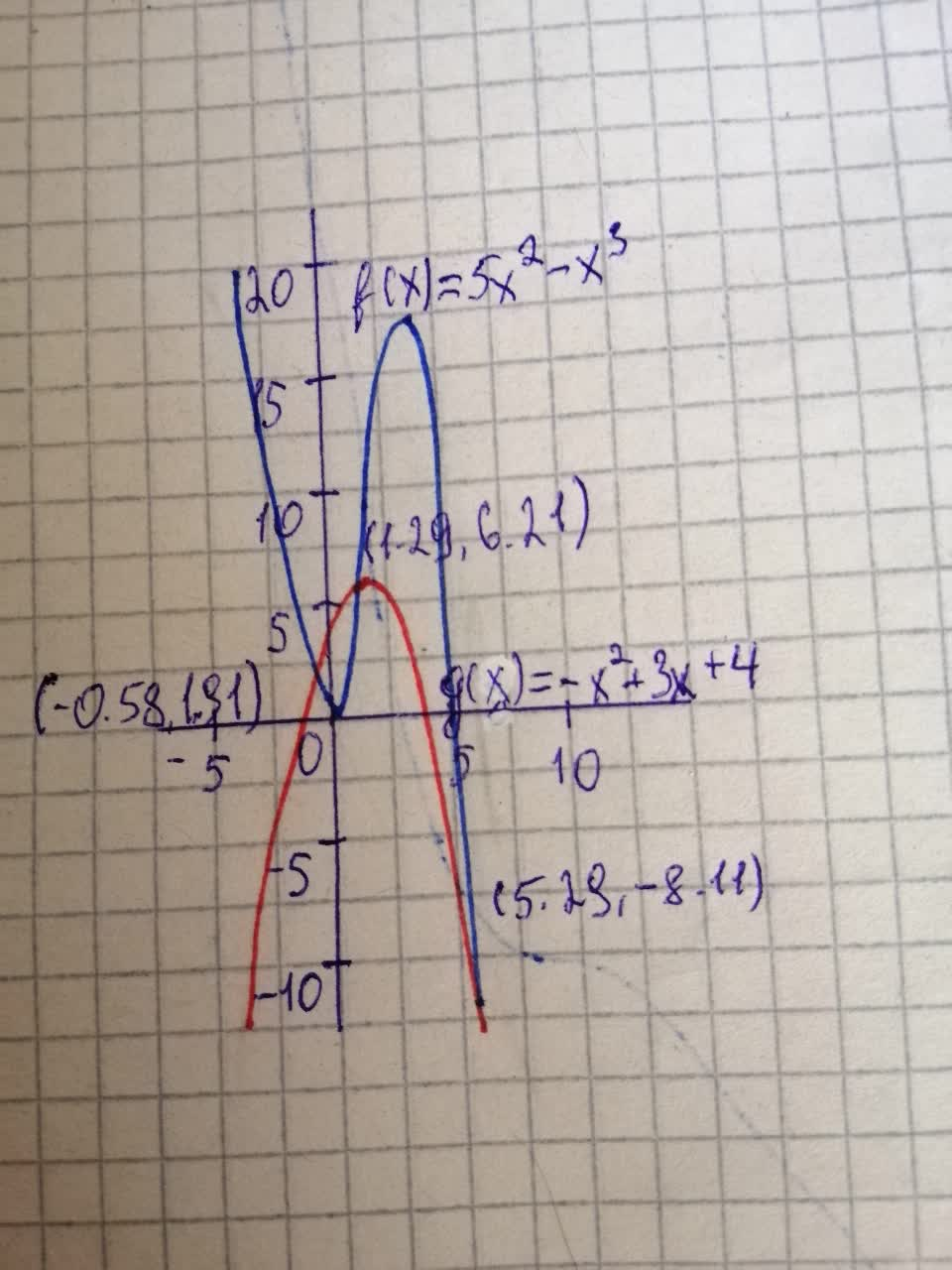Question# Graphically Solve the given equation or inequality graphically 5x^{2}-x^{3}=-x^{2}+3x+4 and 5x^{2}-x^{3}\leq-x^{2}+3x+4

Decimals
ANSWEREDSolving Equations and Inequalities Graphically Solve the given equation or inequality graphically. State your answers rounded to two decimals.
a) $$\displaystyle{5}{x}^{{{2}}}-{x}^{{{3}}}=-{x}^{{{2}}}+{3}{x}+{4}$$
b) $$\displaystyle{5}{x}^{{{2}}}-{x}^{{{3}}}\leq-{x}^{{{2}}}+{3}{x}+{4}$$2021-08-05
Step 1
a) Definition: Solving Equations Graphically
The solution(s) of the equation $$\displaystyle{f{{\left({x}\right)}}}={g{{\left({x}\right)}}}$$ are the values of x where the graphs of f and g intersect.
Step 2
Observation:
The provided equation is $$\displaystyle{5}{x}^{{{2}}}-{x}^{{{3}}}=-{x}^{{{2}}}+{3}{x}+{4}$$
From the definition, it can be directly consider that $$\displaystyle{f{{\left({x}\right)}}}={5}{x}^{{{2}}}-{x}^{{{3}}}$$ and $$\displaystyle{\left({x}\right)}=-{x}^{{{2}}}+{3}{x}+{4}$$
By the definition of solving equation, find the intersection of functions f and g bby graphing it to get the solution.
Let's graph the function to get the intersection.It can be observed from the above graph that the functions $$\displaystyle{f{{\left({x}\right)}}}$$ and $$\displaystyle{g{{\left({x}\right)}}}$$ are intersecting at the points $$\displaystyle{x}=-{0.58},\ {x}={1.29}$$ and $$\displaystyle{x}={5.29}$$
Step 3
b) Definition: Solving Equations Graphically
The solution(s) of the inequality $$\displaystyle{f{{\left({x}\right)}}}\geq\ {g{{\left({x}\right)}}}$$ are the values of x where the graphs of g is higher that the graph of f.
Interpretation:
The provided inequality is $$\displaystyle{5}{x}^{{{2}}}-{x}^{{{3}}}\leq-{x}^{{{2}}}+{3}{x}+{4}$$
From the definition, it can be directly consider that $$\displaystyle{f{{\left({x}\right)}}}={5}{x}^{{{2}}}-{x}^{{{3}}}$$ and $$\displaystyle{\left({x}\right)}=-{x}^{{{2}}}+{3}{x}+{4}$$
By the definition of solving inequality, find the f lower than that of g.
It can be observed from graph of part (a) that the function $$\displaystyle{f{{\left({x}\right)}}}$$ is lower than $$\displaystyle{g{{\left({x}\right)}}}$$ between $$\displaystyle-{0.58}$$ to $$\displaystyle{1.29}$$ and between the range $$\displaystyle{5.29}$$ to $$\displaystyle\infty$$ Therefore, from the point $$\displaystyle{x}=-{0.58}$$ to $$\displaystyle{x}={1.29}$$ and from $$\displaystyle{x}={5.29}$$ to $$\displaystyle{x}=\infty$$ the function $$\displaystyle{f{{\left({x}\right)}}}$$ is lower than or equal to $$\displaystyle{g{{\left({x}\right)}}}$$.